# Theoretical Diesel Engine Cycle, Performance and Diagram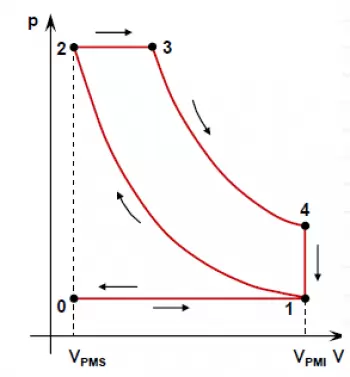The theoretical diesel cycle is the theoretical cycle of a diesel engine, also known as a compression ignition engine.

The theoretical cycle of a heat engine is a theoretical approximation of its operation to calculate its performance.

The cycle of an internal combustion engine is constituted by the physical and chemical transformations that the fuel undergoes during its passage inside the engine.

The study of a real cycle, taking into account all the numerous variables, represents a very complex problem. For this reason, it is commonly simplified by resorting to theoretical approaches, based on different simplified assumptions.

The fundamental difference between the Otto cycle and the diesel cycle is in the heat introduction phase. In the Otto cycle, heat is introduced at constant volume, while in the diesel cycle it is carried out at constant pressure. Another difference between the two cycles lies in the values ​​of the compression ratio, which varies from 12 to 22 for the diesel engine, while it ranges only between 6 and 10 for the Otto engine or gasoline engines.

## Theoretical Cycle Diagram of a Diesel Engine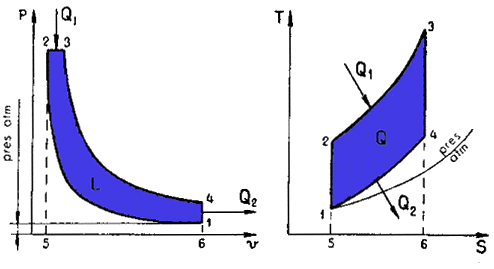As seen in the figure, the ideal diesel cycle is made up of four thermal lines that represent:

• Adiabatic compression. No heat exchange. (1-2);
• Introduction of heat at constant pressure (2-3);
• Adiabatic expansion. No heat exchange (3-4);
• Constant volume heat expulsion (4-1).

## What Is the Theoretical Cycle Performance of a Diesel Engine?

During the 2-3 transformation of the introduction of heat Q1 at constant pressure, the piston goes into operation, and therefore, the fluid produces the work: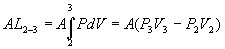Therefore, the energy equation without flow becomes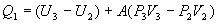and the enthalpy h of the fluid is given by the expression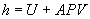The equation becomes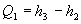Since the fluid is a perfect gas, we can use, for its enthalpy variation at constant pressure, the expression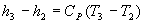Then the heat entered will have the following value:It should be noted that in a transformation with the introduction of heat at constant pressure, the value of the enthalpy of the active fluid varies, while in the case of a transformation at constant volume, the value of the internal energy of the fluid varies. Since the heat subtraction Q2 is carried out as in the Otto cycle, we can write:

Q 2 = U 4 -U 1

and since the fluid is a perfect gas and the cycle is ideal:

Q 2 = C v (T 4 -T 1 ).

Therefore, the ideal thermal performance of the theoretical diesel cycle is:

h e = (heat supplied - heat subtracted) / heat supplied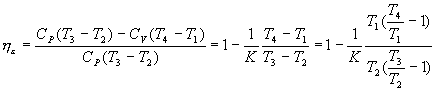expression completely analogous to that found for the ideal performance of the theoretical Otto cycle.

For the 2-3 transformation of constant pressure combustion we have: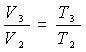For the adiabatic transformations 1-2 compression and 3-4 expansion we have, respectively: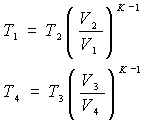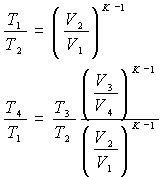from where:

and since they are V 4 = V 1   and T 3 / T 2 = V 3 / V 2   , we can write: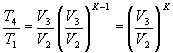Substituting this expression for the ideal thermal performance, it results: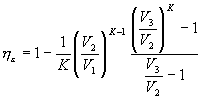indicating with t 'the relationship between the volumes V 3  and V 2  at the end and at the beginning, respectively, of the constant pressure combustion phase, to which we will give the name "constant pressure combustion ratio", and recalling that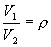we finally obtain the expression of the  ideal thermal efficiency of the theoretical cycle say l: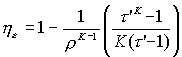In this expression we see that he is, for the diesel cycle, a function of the compression ratio, the constant pressure combustion ratio and the ratio k between the specific heats.

The expressions of the thermal efficiencies of the Otto and diesel cycles differ only by the term in parentheses, which is always greater than 1, and, therefore, it is clear that with equal compression ratio he is greater for the Otto cycle than for the diesel cycle. By reducing t ', that is, the heat introduced at constant pressure, the performance he of the diesel cycle approaches that of the Otto cycle, with which it matches for t' = 1.

Author:

Published: December 10, 2009
Last review: April 14, 2018